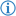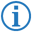The greater than or equal operator is a type of comparison operator which returns the boolean value `<literal:true>` if the first number object's value is bigger than the second's or equal to it, else returns `<literal:false>`.

Its token is named GREATER_THAN_OR_EQUAL.

## Syntax

### List of Related Phrases• `had no less than`
• `has no less than`
• `is no less than`
• `is not less than`
• `isn't less than`
• `was no less than`
• `was not less than`
• `wasn't less than`
• `were no less than`
• `were not less than`
• `weren't less than`

### FDS### Example

`If`` ``Spike's age`` ``wasn't less than`` ``14`` ``then``:`

This executes the next part of code only if the variable `Spike's age` is bigger than `14` or equal to it.

This is equivalent to the following Java code:

```if(Spike_s_age>=8) {
```